# Quantification of Elastic Modulus in Materials Using AFM

The invention of atomic force microscopy (AFM) was a revolutionary moment in the world of the material sciences and in device engineering. The technique has made accurate, reliable and non-destructive imaging possible at the nanoscale. AFM can be used in numerous nanotechnology applications, ranging from biomedical implantable actuators to ultrathin cathode material for batteries, as well as in photo detectors and switches for memory storage and logic circuits [1-3].

The dimensions of the device are flexible – they can shrink continuously, which has made the measurement of local properties of materials more effective in bulk, providing accurate nanoscale measurement. Critical parameters that determine how reliable the devices are and how well they perform include local mechanical properties, such as adhesion and elastic modulus [4-6].

Existing AFM-based nanomechanical methods, such as force-volume spectroscopy and nanoindentation, have been introduced to measure mechanical properties. Nevertheless, some of these techniques take a long time to be conveyed, whereas others are destructive. This can make in situ and high-throughput monitoring in certain applications quite difficult.

Figure 1 shows principles of AFM PinPoint™ nanomechanical mode developed by Park Systems. The PinPoint™ technique is patented and is at least two orders of magnitude faster than conventional force-volume techniques. This allows the user to obtain quantitative mechanical properties and high-resolution topography images of materials simultaneously over a short period of time.

The moving of the tip - in an approach-retract manner - eliminates any lateral force. This is possible during operation, and it also ensures a frictionless operation. The frictionless operation, in particular, is what eliminates the lateral force due to the constant contact between tip and sample. The tip and the sample condition are, thus, preserved, and this provides ideal measurement for both hard and soft samples. The sample may include hard disks, but also biological samples.

Force-distance curves can be acquired at each point of the stage, which can then be used to calculate the mechanical characteristics of the sample being measured. While the data acquisition is ongoing, the XY scanner stops and the contact time is controlled to allow enough time for the scanner to acquire precise and accurate data.

In the following experiment, four different materials with various ranges of modulus were successfully quantified. The results acquired for each test are close to the nominal modulus value of each material. This proves that the PinPoint™ mode is superior in its ability to quantify mechanical properties. Additionally, the features that the surface possesses have been revealed by simultaneously-acquired high-resolution images obtained.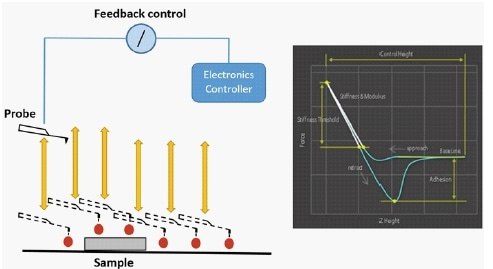Figure 1. Principles of PinPoint’s™ nanomechanical mode by Park Systems. This diagram demonstrates the feedback-controlled approach and retraction of a probe at multiple sites along a sample’s surface. Feedback control from the AFM systems’ controller allows this technique to acquire both surface topography. The F/D curve demonstrates how Pinpoint extracts mechanical property data.

## Experimental

### Sample and Tip

The Highly Ordered Pyrolytic Graphic (HOPG), Polydimethylsiloxane (PDMS) and Polystyrene – low-density polyolefin elastomer (PS-LDPE) were measured with an Olympus micro cantilever (OMCL-AC160TS) with nominal spring constant k = 26 N/m and resonant frequency f = 300 kHz.

A high degree of crystallographic orientation is achieved with the annealing of the HOPG sample (a highly ordered form of highly pure Pyrolytic) under high temperatures and pressure. The PDMS sample is a silicone polymer, which is physically soft, like rubber or resin, and is mounted on a magnetic sample disk. The PS-LDPE sample is a copolymer composed of PS and PE that was spin-cast onto a silicon substrate, creating a film with different modulus properties. PS serves as the matrix, while PE is the low-density doping component .

### Pinpoint Imaging Conditions

The samples were investigated using the Park NX20 AFM system under ambient air condition. Except for scan range, the scanned parameters used in measuring the samples were all the same.

## Results and Discussion

### Hertzian model

The mechanical properties of Pinpoint mode, such as elastic modulus, can be quantified based on the well-established Hertzian model. In this technique, it is assumed that no other interaction than elastic deformation can be observed between tip and sample. The calculation is based on the equation E (Elastic modulus) = F (Loading force). The geometries of the tip influence greatly the equation. Assuming that a spherical tip with a specific radius (R) is being used to indent elastic half space to a displacement of d, the applied force can be derived as below: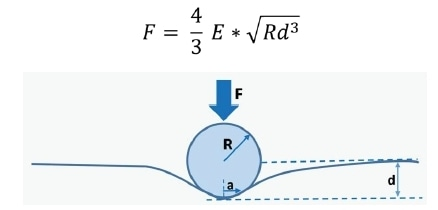Figure 2. Illustration of spherical tip and sample interaction showing the displacement created in the sample surface as force is applied.

The measurement of the corresponding F, R, and d, the E* can also be reversed-calculated. However, E* is a function of two materials, and its equation can be expressed as below: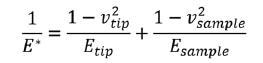Etip and Esample are the elastic modulus of the two materials and νtip and νsample are their corresponding Poisson's ratio. By knowing E*, Etip, νtip and νsample, Esample can be reversed-calculated .

Topography images and the corresponding maps of elastic modulus acquired during the tests are shown in Table 1. XEI software - developed by Park Systems - was used to analyze the images from the experiment, mapping the acquired signals to a color table.

For topography images, the intensity of the shading correlates to the surface height variation, with extremely bright and dark areas having the highest and lowest height regions, respectively. The same metric applies with the elastic modulus, wherein brighter and darker regions correspond to areas with higher and lower elastic modulus.

The topography image of HOPG indicated that sample surfaces are made of atomic terraces with step edges. These terraces demonstrate a homogenous diagonal path and were measured to have approximately 1.5 nm step height.

On the contrary, the elastic modulus map shows only a few variations on the mechanical properties of the sample surfaces. The diagonal dark lines visible on the elastic modulus image are representative of a surface with low modulus.

The topography image of the PDMS revealed the rough surface of the sample. Both topography and modulus image shows that there are only minor variations in the height and modulus of the surfaces. The topography image of PS-LDPE confirms that the sample consists of two different materials. The dark circular dots features are the LDPE (low-density polyolefin) materials, while the brighter regions in this image are the PS (polystyrene) materials.

As anticipated, there are two domains that the elastic modulus map includes, wherein LDPE materials (represented by dark circular dots) are the domains with lower modulus, while PS (represented by areas with bright color) are the domains with a higher modulus.

Table 1. Topography image and correlated maps of elastic modulus acquired for each material.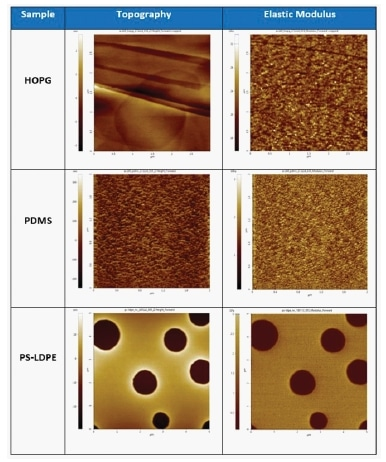Tables 2 and 3 show the quantitative results of elastic and the corresponding values on a graph. The results were plotted to better analyze the data. In order to evaluate the performance of Pinpoint mode, the results acquired in this experiment were compared with the nominal values of each sample. The result of this comparison showed that the measured modulus values of all the samples are close to their nominal values.

For HOPG, the measured modulus is 22.09 GPa, which is close to its nominal value of 18 GPa. For PDMS, the measured modulus is 0.0018 GPa, close to its nominal value of 0.003 GPa. While for PS-LDPE, the measured values are1.955 GPa and 0.132 GPa, which are also comparable to their nominal values of 2 GPa and 0.1 GPa, respectively. The standard deviation of the modulus results was also calculated and based on the results, PDMS sample has the lowest standard deviation with Std = 4.03E-04 GPa, while the HOPG sample has the highest standard deviation with Std =2.224 GPa.

Table 2. Measured mean and standard deviation (Std) of elastic modulus.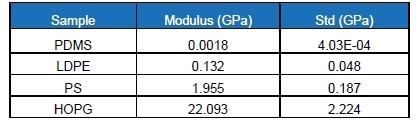Table 3. Plotted value of measured mean and standard deviation of elastic modulus.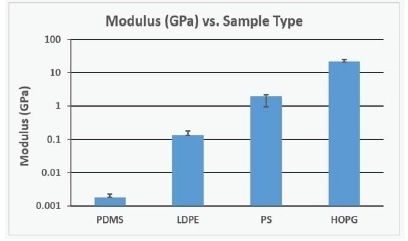## Conclusion

Using the PinPoint™ nanomechanical mode, the Park NX20 AFM managed to successfully acquire quantitative and qualitative data of the four materials. The surface features of the samples, such as the atomic terraces surface of the HOPG, the rough surface of the PDMS, and the circular dots matrix of the PS-LDPE were observable in the surface features of the samples.

The measured elastic modulus of each sample was close to their nominal modulus value. This confirmed that the PinPoint™ nanomechanical mode is capable of quantifying a large number of mechanical properties of different materials and can provide researchers with accurate material surface information.

1. Dr. R. Cook, Nanomechanical Measurements and Tools, Written by AZoNanoJan 17 2010.
2. F. Wu, et al., Ultrathin Spinel Membrane-Encapsulated Layered Lithium-Rich Cathode Material for Advanced Li-Ion Batteries, Nano Lett., 2014, 14 (6), pp 3550–3555.
3. H. Kind, et al., Nanowire Ultraviolet Photodetectors and OpticalSwitches, Advanced Materials.
4. Z. Drira, et al., Nanomechanical measurements of polyethylene glycol hydrogels using atomic force microscopy, Volume 18, February 2013, Pages 20-28.
5. J. Pineda, et al., Optimum Current Distribution Measurement of Zinc Oxide Nanorods via PinPoint™ Conductive AFM.
6. C. Clifford, et al., Quantification issues in the identification of nanoscale regions of homopolymers using modulus measurement via AFM nanoindentation, Applied Surface Science, Volume 252, Issue 5, 15 December 2005, Pages 1915-1933.
7. M. Hong, et al., Collagen Fibrils Imaging in Air and in Liquid Using Atomic Force Microscope-Based Fast Nanomechanical Mode.
8. W. Shi, et al., PinPoint Nanomechanical Mode Using Probes with Different Stiffness.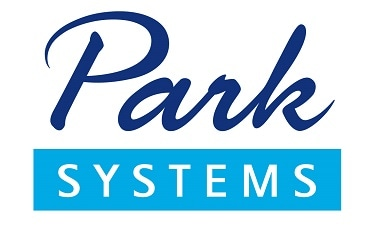This information has been sourced, reviewed and adapted from materials provided by Park Systems Inc.

## Citations

• APA

Park Systems. (2019, November 22). Quantification of Elastic Modulus in Materials Using AFM. AZoNano. Retrieved on August 08, 2020 from https://www.azonano.com/article.aspx?ArticleID=5055.

• MLA

Park Systems. "Quantification of Elastic Modulus in Materials Using AFM". AZoNano. 08 August 2020. <https://www.azonano.com/article.aspx?ArticleID=5055>.

• Chicago

Park Systems. "Quantification of Elastic Modulus in Materials Using AFM". AZoNano. https://www.azonano.com/article.aspx?ArticleID=5055. (accessed August 08, 2020).

• Harvard

Park Systems. 2019. Quantification of Elastic Modulus in Materials Using AFM. AZoNano, viewed 08 August 2020, https://www.azonano.com/article.aspx?ArticleID=5055.